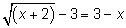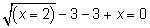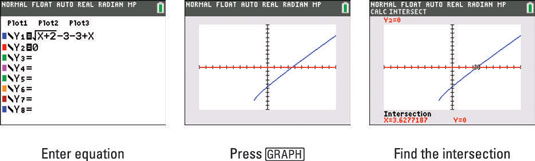##### TI-84 Plus CE Graphing Calculator For Dummies, 3rd EditionThere are a number of different ways to solve an equation by graphing on your TI-84 Plus calculator. The basic idea is to set the equation equal to zero, graph it, and find the zeros. This method has a twist.

To solve the equation,1. Set your equation equal to zero.

Subtract 3 and add x to both sides:2. Press [Y=] and enter one side of your equation in Y1 and enter the other side of your equation in Y2.

See the first screen. Notice, one of your functions is a horizontal line on the x-axis. It's easier to find intersection points than it is to find zeros. You might be wondering what the twist is with this method? When you find an intersection point of the two graphs, you have effectively found a zero of the equation! Pretty nifty, huh?3. Press [GRAPH] and graph your functions in a viewing window that contains the intersection points of the two functions.

See the second screen. You may need to zoom out by pressing [ZOOM] and pressing [ENTER] to make sure you have all the intersection points in the graphing window.

4. Press [2nd][TRACE][ENTER][ENTER][ENTER] to find one of the intersection point(s) of the two graphed functions.

See the third screen. If there is more than one intersection point, you must press [2nd][TRACE][ENTER][ENTER] and use the right- and left-arrow keys to navigate near the other intersection point. Press [ENTER] to make your guess.

You find your solution in the border at the bottom of the screen beneath the word “Intersection.”